Anzeige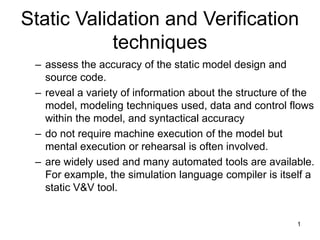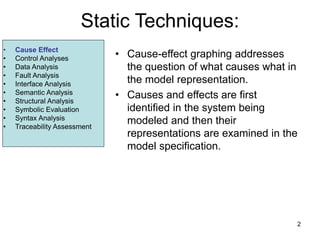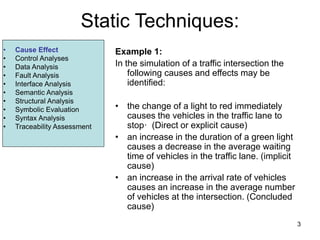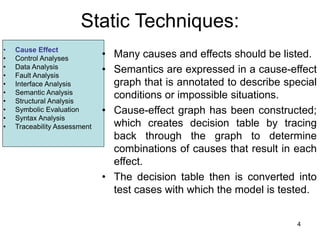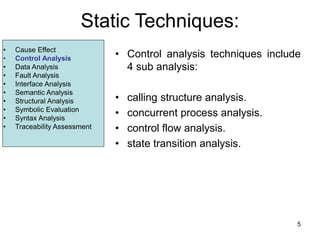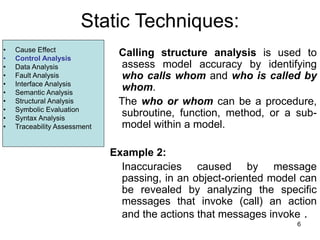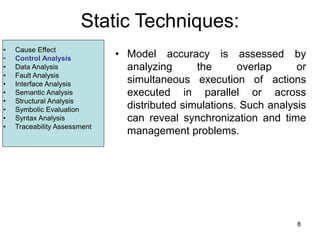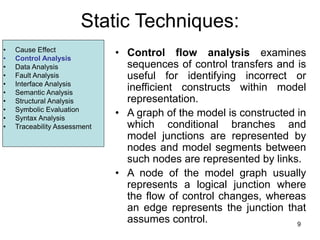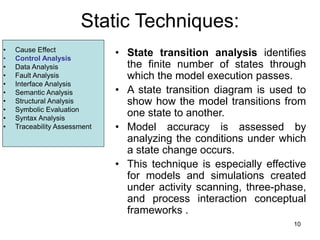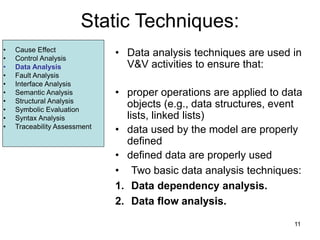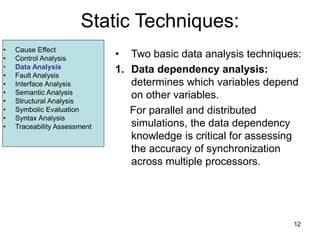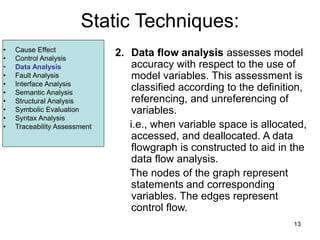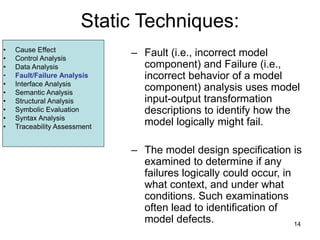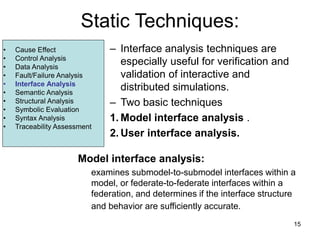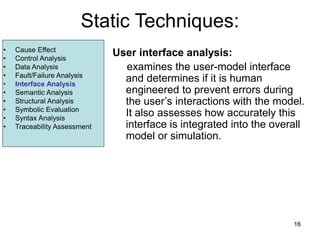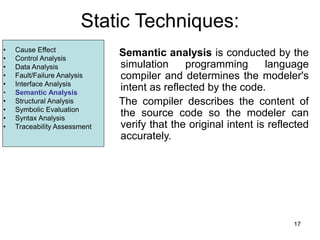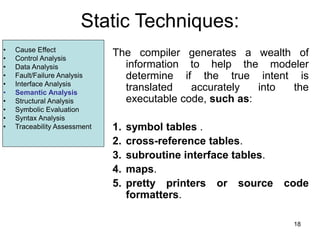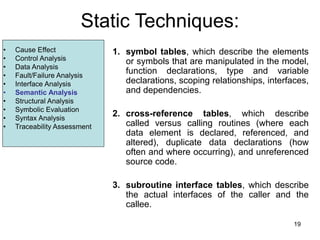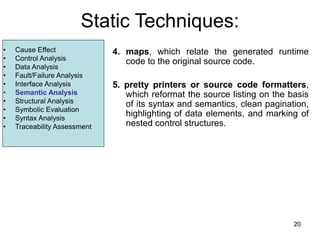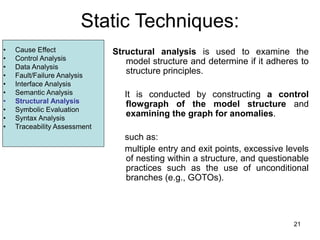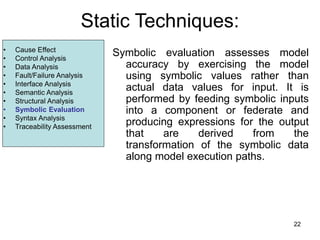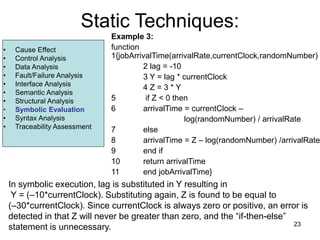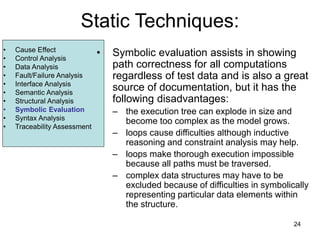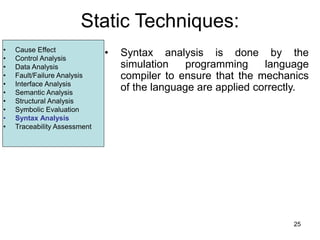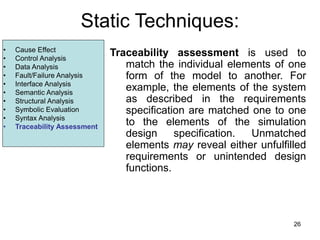1 von 26
Anzeige

### Software_Validation_and_Verification-Static_techniques-.ppt

1. 1 Static Validation and Verification techniques – assess the accuracy of the static model design and source code. – reveal a variety of information about the structure of the model, modeling techniques used, data and control flows within the model, and syntactical accuracy – do not require machine execution of the model but mental execution or rehearsal is often involved. – are widely used and many automated tools are available. For example, the simulation language compiler is itself a static V&V tool.
2. 2 Static Techniques: • Cause-effect graphing addresses the question of what causes what in the model representation. • Causes and effects are first identified in the system being modeled and then their representations are examined in the model specification. • Cause Effect • Control Analyses • Data Analysis • Fault Analysis • Interface Analysis • Semantic Analysis • Structural Analysis • Symbolic Evaluation • Syntax Analysis • Traceability Assessment
3. 3 Static Techniques: • Cause Effect • Control Analyses • Data Analysis • Fault Analysis • Interface Analysis • Semantic Analysis • Structural Analysis • Symbolic Evaluation • Syntax Analysis • Traceability Assessment Example 1: In the simulation of a traffic intersection the following causes and effects may be identified: • the change of a light to red immediately causes the vehicles in the traffic lane to stop· (Direct or explicit cause) • an increase in the duration of a green light causes a decrease in the average waiting time of vehicles in the traffic lane. (implicit cause) • an increase in the arrival rate of vehicles causes an increase in the average number of vehicles at the intersection. (Concluded cause) • Cause Effect • Control Analyses • Data Analysis • Fault Analysis • Interface Analysis • Semantic Analysis • Structural Analysis • Symbolic Evaluation • Syntax Analysis • Traceability Assessment
4. 4 Static Techniques: • Many causes and effects should be listed. • Semantics are expressed in a cause-effect graph that is annotated to describe special conditions or impossible situations. • Cause-effect graph has been constructed; which creates decision table by tracing back through the graph to determine combinations of causes that result in each effect. • The decision table then is converted into test cases with which the model is tested. • Cause Effect • Control Analyses • Data Analysis • Fault Analysis • Interface Analysis • Semantic Analysis • Structural Analysis • Symbolic Evaluation • Syntax Analysis • Traceability Assessment
5. 5 Static Techniques: • Control analysis techniques include 4 sub analysis: • calling structure analysis. • concurrent process analysis. • control flow analysis. • state transition analysis. • Cause Effect • Control Analysis • Data Analysis • Fault Analysis • Interface Analysis • Semantic Analysis • Structural Analysis • Symbolic Evaluation • Syntax Analysis • Traceability Assessment
6. 6 Static Techniques: Calling structure analysis is used to assess model accuracy by identifying who calls whom and who is called by whom. The who or whom can be a procedure, subroutine, function, method, or a sub- model within a model. Example 2: Inaccuracies caused by message passing, in an object-oriented model can be revealed by analyzing the specific messages that invoke (call) an action and the actions that messages invoke . • Cause Effect • Control Analysis • Data Analysis • Fault Analysis • Interface Analysis • Semantic Analysis • Structural Analysis • Symbolic Evaluation • Syntax Analysis • Traceability Assessment
7. 7 Static Techniques: Concurrent process analysis is especially useful for parallel and distributed simulations. • a simulation executing on a single computer with a single processor (CPU) is referred to as a serial (sequential) simulation. • a simulation executing on a single computer with multiple processors is a parallel simulation. • a simulation executed on multiple single-processor computers is said to be a distributed simulation. • Cause Effect • Control Analysis • Data Analysis • Fault Analysis • Interface Analysis • Semantic Analysis • Structural Analysis • Symbolic Evaluation • Syntax Analysis • Traceability Assessment
8. 8 Static Techniques: • Model accuracy is assessed by analyzing the overlap or simultaneous execution of actions executed in parallel or across distributed simulations. Such analysis can reveal synchronization and time management problems. • Cause Effect • Control Analysis • Data Analysis • Fault Analysis • Interface Analysis • Semantic Analysis • Structural Analysis • Symbolic Evaluation • Syntax Analysis • Traceability Assessment
9. 9 Static Techniques: • Control flow analysis examines sequences of control transfers and is useful for identifying incorrect or inefficient constructs within model representation. • A graph of the model is constructed in which conditional branches and model junctions are represented by nodes and model segments between such nodes are represented by links. • A node of the model graph usually represents a logical junction where the flow of control changes, whereas an edge represents the junction that assumes control. • Cause Effect • Control Analysis • Data Analysis • Fault Analysis • Interface Analysis • Semantic Analysis • Structural Analysis • Symbolic Evaluation • Syntax Analysis • Traceability Assessment
10. 10 Static Techniques: • State transition analysis identifies the finite number of states through which the model execution passes. • A state transition diagram is used to show how the model transitions from one state to another. • Model accuracy is assessed by analyzing the conditions under which a state change occurs. • This technique is especially effective for models and simulations created under activity scanning, three-phase, and process interaction conceptual frameworks . • Cause Effect • Control Analysis • Data Analysis • Fault Analysis • Interface Analysis • Semantic Analysis • Structural Analysis • Symbolic Evaluation • Syntax Analysis • Traceability Assessment
11. 11 Static Techniques: • Data analysis techniques are used in V&V activities to ensure that: • proper operations are applied to data objects (e.g., data structures, event lists, linked lists) • data used by the model are properly defined • defined data are properly used • Two basic data analysis techniques: 1. Data dependency analysis. 2. Data flow analysis. • Cause Effect • Control Analysis • Data Analysis • Fault Analysis • Interface Analysis • Semantic Analysis • Structural Analysis • Symbolic Evaluation • Syntax Analysis • Traceability Assessment
12. 12 Static Techniques: • Two basic data analysis techniques: 1. Data dependency analysis: determines which variables depend on other variables. For parallel and distributed simulations, the data dependency knowledge is critical for assessing the accuracy of synchronization across multiple processors. • Cause Effect • Control Analysis • Data Analysis • Fault Analysis • Interface Analysis • Semantic Analysis • Structural Analysis • Symbolic Evaluation • Syntax Analysis • Traceability Assessment
13. 13 Static Techniques: 2. Data flow analysis assesses model accuracy with respect to the use of model variables. This assessment is classified according to the definition, referencing, and unreferencing of variables. i.e., when variable space is allocated, accessed, and deallocated. A data flowgraph is constructed to aid in the data flow analysis. The nodes of the graph represent statements and corresponding variables. The edges represent control flow. • Cause Effect • Control Analysis • Data Analysis • Fault Analysis • Interface Analysis • Semantic Analysis • Structural Analysis • Symbolic Evaluation • Syntax Analysis • Traceability Assessment
14. 14 Static Techniques: – Fault (i.e., incorrect model component) and Failure (i.e., incorrect behavior of a model component) analysis uses model input-output transformation descriptions to identify how the model logically might fail. – The model design specification is examined to determine if any failures logically could occur, in what context, and under what conditions. Such examinations often lead to identification of model defects. • Cause Effect • Control Analysis • Data Analysis • Fault/Failure Analysis • Interface Analysis • Semantic Analysis • Structural Analysis • Symbolic Evaluation • Syntax Analysis • Traceability Assessment
15. 15 Static Techniques: – Interface analysis techniques are especially useful for verification and validation of interactive and distributed simulations. – Two basic techniques 1. Model interface analysis . 2. User interface analysis. Model interface analysis: examines submodel-to-submodel interfaces within a model, or federate-to-federate interfaces within a federation, and determines if the interface structure and behavior are sufficiently accurate. • Cause Effect • Control Analysis • Data Analysis • Fault/Failure Analysis • Interface Analysis • Semantic Analysis • Structural Analysis • Symbolic Evaluation • Syntax Analysis • Traceability Assessment
16. 16 Static Techniques: User interface analysis: examines the user-model interface and determines if it is human engineered to prevent errors during the user’s interactions with the model. It also assesses how accurately this interface is integrated into the overall model or simulation. • Cause Effect • Control Analysis • Data Analysis • Fault/Failure Analysis • Interface Analysis • Semantic Analysis • Structural Analysis • Symbolic Evaluation • Syntax Analysis • Traceability Assessment
17. 17 Static Techniques: Semantic analysis is conducted by the simulation programming language compiler and determines the modeler's intent as reflected by the code. The compiler describes the content of the source code so the modeler can verify that the original intent is reflected accurately. • Cause Effect • Control Analysis • Data Analysis • Fault/Failure Analysis • Interface Analysis • Semantic Analysis • Structural Analysis • Symbolic Evaluation • Syntax Analysis • Traceability Assessment
18. 18 Static Techniques: The compiler generates a wealth of information to help the modeler determine if the true intent is translated accurately into the executable code, such as: 1. symbol tables . 2. cross-reference tables. 3. subroutine interface tables. 4. maps. 5. pretty printers or source code formatters. • Cause Effect • Control Analysis • Data Analysis • Fault/Failure Analysis • Interface Analysis • Semantic Analysis • Structural Analysis • Symbolic Evaluation • Syntax Analysis • Traceability Assessment
19. 19 Static Techniques: 1. symbol tables, which describe the elements or symbols that are manipulated in the model, function declarations, type and variable declarations, scoping relationships, interfaces, and dependencies. 2. cross-reference tables, which describe called versus calling routines (where each data element is declared, referenced, and altered), duplicate data declarations (how often and where occurring), and unreferenced source code. 3. subroutine interface tables, which describe the actual interfaces of the caller and the callee. • Cause Effect • Control Analysis • Data Analysis • Fault/Failure Analysis • Interface Analysis • Semantic Analysis • Structural Analysis • Symbolic Evaluation • Syntax Analysis • Traceability Assessment
20. 20 Static Techniques: 4. maps, which relate the generated runtime code to the original source code. 5. pretty printers or source code formatters, which reformat the source listing on the basis of its syntax and semantics, clean pagination, highlighting of data elements, and marking of nested control structures. • Cause Effect • Control Analysis • Data Analysis • Fault/Failure Analysis • Interface Analysis • Semantic Analysis • Structural Analysis • Symbolic Evaluation • Syntax Analysis • Traceability Assessment
21. 21 Static Techniques: Structural analysis is used to examine the model structure and determine if it adheres to structure principles. It is conducted by constructing a control flowgraph of the model structure and examining the graph for anomalies. such as: multiple entry and exit points, excessive levels of nesting within a structure, and questionable practices such as the use of unconditional branches (e.g., GOTOs). • Cause Effect • Control Analysis • Data Analysis • Fault/Failure Analysis • Interface Analysis • Semantic Analysis • Structural Analysis • Symbolic Evaluation • Syntax Analysis • Traceability Assessment
22. 22 Static Techniques: Symbolic evaluation assesses model accuracy by exercising the model using symbolic values rather than actual data values for input. It is performed by feeding symbolic inputs into a component or federate and producing expressions for the output that are derived from the transformation of the symbolic data along model execution paths. • Cause Effect • Control Analysis • Data Analysis • Fault/Failure Analysis • Interface Analysis • Semantic Analysis • Structural Analysis • Symbolic Evaluation • Syntax Analysis • Traceability Assessment
23. 23 Static Techniques: Example 3: function 1{jobArrivalTime(arrivalRate,currentClock,randomNumber) 2 lag = -10 3 Y = lag * currentClock 4 Z = 3 * Y 5 if Z < 0 then 6 arrivalTime = currentClock – log(randomNumber) / arrivalRate 7 else 8 arrivalTime = Z – log(randomNumber) /arrivalRate 9 end if 10 return arrivalTime 11 end jobArrivalTime} • Cause Effect • Control Analysis • Data Analysis • Fault/Failure Analysis • Interface Analysis • Semantic Analysis • Structural Analysis • Symbolic Evaluation • Syntax Analysis • Traceability Assessment In symbolic execution, lag is substituted in Y resulting in Y = (–10*currentClock). Substituting again, Z is found to be equal to (–30*currentClock). Since currentClock is always zero or positive, an error is detected in that Z will never be greater than zero, and the “if-then-else” statement is unnecessary.
24. 24 Static Techniques: • Symbolic evaluation assists in showing path correctness for all computations regardless of test data and is also a great source of documentation, but it has the following disadvantages: – the execution tree can explode in size and become too complex as the model grows. – loops cause difficulties although inductive reasoning and constraint analysis may help. – loops make thorough execution impossible because all paths must be traversed. – complex data structures may have to be excluded because of difficulties in symbolically representing particular data elements within the structure. • Cause Effect • Control Analysis • Data Analysis • Fault/Failure Analysis • Interface Analysis • Semantic Analysis • Structural Analysis • Symbolic Evaluation • Syntax Analysis • Traceability Assessment
25. 25 Static Techniques: • Syntax analysis is done by the simulation programming language compiler to ensure that the mechanics of the language are applied correctly. • Cause Effect • Control Analysis • Data Analysis • Fault/Failure Analysis • Interface Analysis • Semantic Analysis • Structural Analysis • Symbolic Evaluation • Syntax Analysis • Traceability Assessment
26. 26 Static Techniques: Traceability assessment is used to match the individual elements of one form of the model to another. For example, the elements of the system as described in the requirements specification are matched one to one to the elements of the simulation design specification. Unmatched elements may reveal either unfulfilled requirements or unintended design functions. • Cause Effect • Control Analysis • Data Analysis • Fault/Failure Analysis • Interface Analysis • Semantic Analysis • Structural Analysis • Symbolic Evaluation • Syntax Analysis • Traceability Assessment
Anzeige